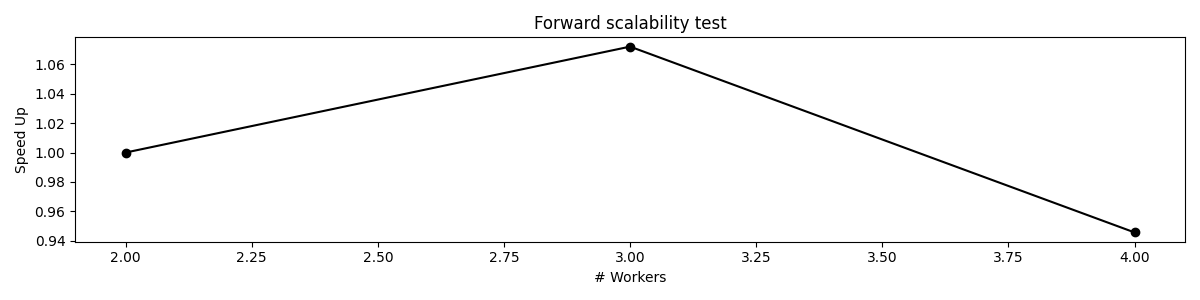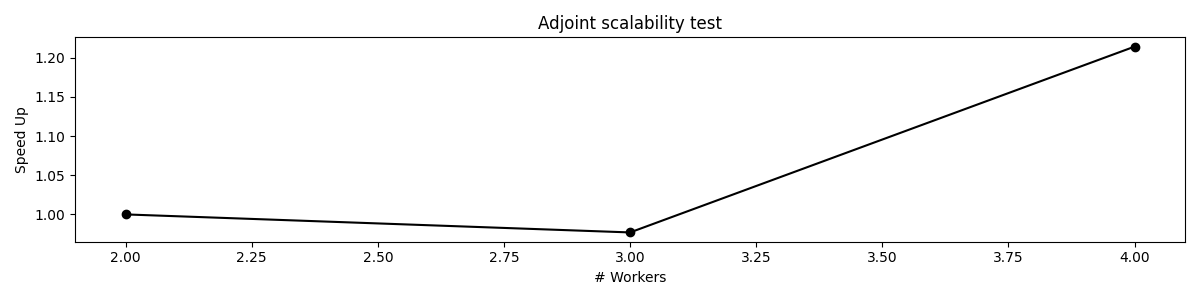# Operators with Multiprocessing¶

This example shows how perform a scalability test for one of PyLops operators that uses multiprocessing to spawn multiple processes. Operators that support such feature are pylops.basicoperators.VStack, pylops.basicoperators.HStack, and pylops.basicoperators.BlockDiagonal, and pylops.basicoperators.Block.

In this example we will consider the BlockDiagonal operator which contains pylops.basicoperators.MatrixMult operators along its main diagonal.

import matplotlib.pyplot as plt
import numpy as np

import pylops

plt.close("all")


Let’s start by creating N MatrixMult operators and the BlockDiag operator

N = 100
Nops = 32
Ops = [pylops.MatrixMult(np.random.normal(0.0, 1.0, (N, N))) for _ in range(Nops)]

Op = pylops.BlockDiag(Ops, nproc=1)


We can now perform a scalability test on the forward operation

workers = [2, 3, 4]
compute_times, speedup = pylops.utils.multiproc.scalability_test(
Op, np.ones(Op.shape), workers=workers, forward=True
)
plt.figure(figsize=(12, 3))
plt.plot(workers, speedup, "ko-")
plt.xlabel("# Workers")
plt.ylabel("Speed Up")
plt.title("Forward scalability test")
plt.tight_layout()Out:

Working with 2 workers...
Working with 3 workers...
Working with 4 workers...


And likewise on the adjoint operation

compute_times, speedup = pylops.utils.multiproc.scalability_test(
Op, np.ones(Op.shape), workers=workers, forward=False
)
plt.figure(figsize=(12, 3))
plt.plot(workers, speedup, "ko-")
plt.xlabel("# Workers")
plt.ylabel("Speed Up")
plt.tight_layout()Out:

Working with 2 workers...
Working with 3 workers...
Working with 4 workers...


Note that we have not tested here the case with 1 worker. In this specific case, since the computations are very small, the overhead of spawning processes is actually dominating the time of computations and so computing the forward and adjoint operations with a single worker is more efficient. We hope that this example can serve as a basis to inspect the scalability of multiprocessing-enabled operators and choose the best number of processes.

Total running time of the script: ( 0 minutes 0.859 seconds)

Gallery generated by Sphinx-Gallery